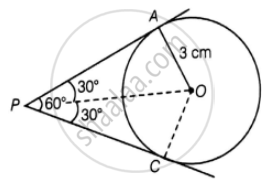# If two tangents inclined at an angle of 60° are drawn to a circle of radius 3 cm the length of each tangent is equal to ______ - Mathematics

MCQ
Fill in the Blanks

If two tangents inclined at an angle of 60° are drawn to a circle of radius 3 cm the length of each tangent is equal to ______

#### Options

• (3sqrt(3))/2  cm

• 6 cm

• 3 cm

• 3sqrt(3) cm

#### Solution

If two tangents inclined at an angle of 60° are drawn to a circle of radius 3 cm the length of each tangent is equal to underline(bb(3sqrt(3)  cm).

Explanation:

Let P be an external point from which pair of tangents are drawn as shown in the figure given below:Join OA and OP

Also, OP is a bisector line of ∠APC.

∠APO = ∠CPO = 30°

OA ⊥ AP

Therefore, in triangle OAP

tan 30° = "OA"/"AP"

1/sqrt3 = 3/"AP"

AP = 3sqrt3 cm

Concept: Number of Tangents from a Point on a Circle
Is there an error in this question or solution?

#### APPEARS IN

NCERT Mathematics Exemplar Class 10
Chapter 9 Circles
Exercise 9.1 | Q 9 | Page 104
Share